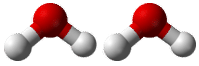# 10.2: Conversions Between Moles and Atoms

Do you hate to type subscripts and superscripts? Even with a good word-processing program, having to click on an icon to get a superscript and then remembering to click off after you type the number can be a real hassle. If we did not know about moles and just knew about numbers of atoms or molecules (those big numbers that require lots of superscripts), life would be much more complicated and we would make many more typing errors.

## Conversions Between Moles and Atoms

### Conversions Between Moles and Number of Particles

Using our unit conversion techniques, we can use the mole label to convert back and forth between the number of particles and moles.

Example $$\PageIndex{1}$$

The element carbon exists in two primary forms: graphite and diamond. How many moles of carbon atoms is $$4.72 \times 10^{24}$$ atoms of carbon?

Solution

Step 1: List the known quantities and plan the problem.

Known

• number of $$\ce{C}$$ atoms $$= 4.72 \times 10^{24}$$
• $$1$$ mole $$= 6.02 \times 10^{23}$$ atoms

Unknown

• $$4.72 \times 10^{24} = ?$$ atoms $$\ce{C}$$

One conversion factor will allow us to convert from the number of $$\ce{C}$$ atoms to moles of $$\ce{C}$$ atoms.

Step 2: Calculate

$4.72 \times 10^{24} \: \text{atoms} \: \ce{C} \times \frac{1 \: \text{mol} \: \ce{C}}{6.02 \times 10^{23} \: \text{atoms} \: \ce{C}} = 7.84 \: \text{mol} \: \ce{C}$

The given number of carbon atoms was greater than Avogadro's number,so the number of moles of $$\ce{C}$$ atoms is greater than 1 mole. Since Avogadro's number is a measured quantity with three significant figures, the result of the calculation is rounded to three significant figures.

Suppose that you wanted to know how many hydrogen atoms were in a mole of water molecules. First, you would need to know the chemical formula for water, which is $$\ce{H_2O}$$. There are two atoms of hydrogen in each molecule of water. How many atoms of hydrogen would there be in two water molecules? There would be $$2 \times 2 = 4$$ hydrogen atoms. How about in a dozen? In that case a dozen is 12 so $$12 \times 2 = 24$$ hydrogen atoms in a dozen water molecules. To get the answers (4 and 24), you had to multiply the given number of molecules by two atoms of hydrogen per molecule. So to find the number of hydrogen atoms in a mole of water molecules, the problem could be solved using conversion factors.

$1 \: \text{mol} \: \ce{H_2O} \times \frac{6.02 \times 10^{23} \: \text{molecules} \: \ce{H_2O}}{1 \: \text{mol} \: \ce{H_2O}} \times \frac{2 \: \text{atoms} \: \ce{H}}{1 \: \text{molecule} \: \ce{H_2O}} = 1.20 \times 10^{24} \: \text{atoms} \: \ce{H}$

The first conversion factor converts from moles of particles to the number of particles. The second conversion factor reflects the number of atoms contained within each molecule.Figure $$\PageIndex{1}$$: Two water molecules contain 4 hydrogen atoms and 2 oxygen atoms. A mole of water molecules contains 2 moles of hydrogen atoms and 1 mole of oxygen atoms.

Example $$\PageIndex{2}$$

Sulfuric acid has the chemical formula $$\ce{H_2SO_4}$$. A certain quantity of sulfuric acid contains $$4.89 \times 10^{25}$$ atoms of oxygen. How many moles of sulfuric acid is the sample?

Solution:

Step 1: List the known quantities and plan the problem.

Known

• $$4.89 \times 10^{25} = \ce{O}$$ atoms
• $$1$$ mole $$= 6.02 \times 10^{23}$$ molecules $$\ce{H_2SO_4}$$

Unknown

• $$\text{mol}$$ of $$\ce{H_2SO_4}$$ molecules

Two conversion factors will be used. First, convert atoms of oxygen to molecules of sulfuric acid. Then, convert molecules of sulfuric acid to moles of sulfuric acid.

Step 2: Calculate

$4.89 \times 10^{25} \: \text{atoms} \: \ce{O} \times \frac{1 \: \text{molecule} \: \ce{H_2SO_4}}{4 \: \text{atoms} \: \ce{O}} \times \frac{1 \: \text{mol} \: \ce{H_2SO_4}}{6.02 \times 10^{23} \: \text{molecules} \: \ce{H_2SO_4}} = 20. \: \text{mol} \: \ce{H_2SO_4}$